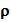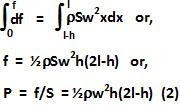Sunday, May 24, 2009

Irodov Problem 1.324As the tube rotates, the centrifugal force (as seen in the rotating frame of the tube) creates a pressure head causing the water to be jetted out of the orifice at the end of the tube.

Let us first compute the pressure at the end of the tube just before the orifice. For this we must consider the centrifugal force exerted by each vertical section of water on the next.

Consider, an infinitesimally thin slice of water of width dx at a distance x from the one end of the tube. Let the area of cross-section of the tube be S. The mass of water contained in this infinitesimally thin slice of water is given by, hereis the density of water. The centrifugal force exerted by this slice of water on the next is given by,The net force acting at the end of the tube just before the orifice can be obtained by integrating over all such thin slices as,In (2) P is the pressure head generated by the centrifugal force acting on the water. The net pressure at the end of the tube just before the orifice is give by P + Po, where Po is the atmospheric pressure. We assume that at this point the water is still i.e. its velocity is zero. Right outside the tube, the water jets into an area of pressure Po (atmospheric pressure), say at a velocity v. Using Bernoulli's equation at points just before and just outside the orifice we have,